# Bubble Sort algorithm using JavaScript

• Difficulty Level : Basic
• Last Updated : 22 Jan, 2021

Bubble sort algorithm is an algorithm that sorts the array by comparing two adjacent elements and swaps them if they are not in the intended order. Here order can be anything like increasing order or decreasing order.

## How Bubble-sort works

We have an unsorted array arr = [ 1, 4, 2, 5, -2, 3 ] the task is to sort the array using bubble sort.

Bubble sort compares the element from index 0 and if the 0th index is less than 1st index then the values get swapped and if the 0th index is less than the 1st index then nothing happens.

then, the 1st index compares to the 2nd index then the 2nd index compares to the 3rd, and so on…

let’s see it with an example, each step is briefly illustrated here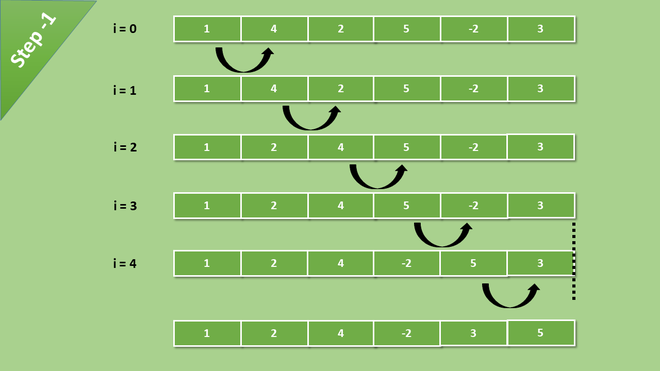Comparisons happen till the last element of the array

After each iteration, the greatest value of the array becomes the last index of the array. in each iteration, the comparison happens till the last unsorted element.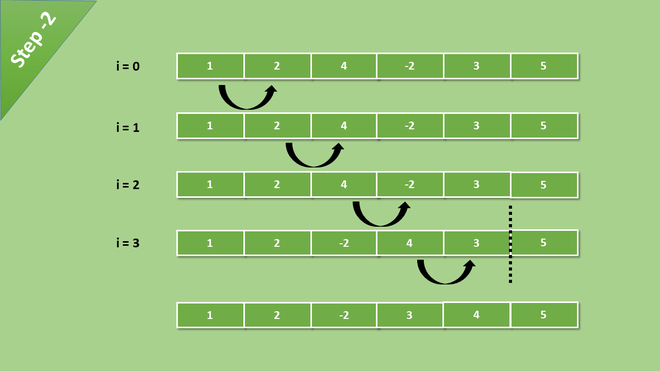Now comparison reduced one step because the biggest element is at its right place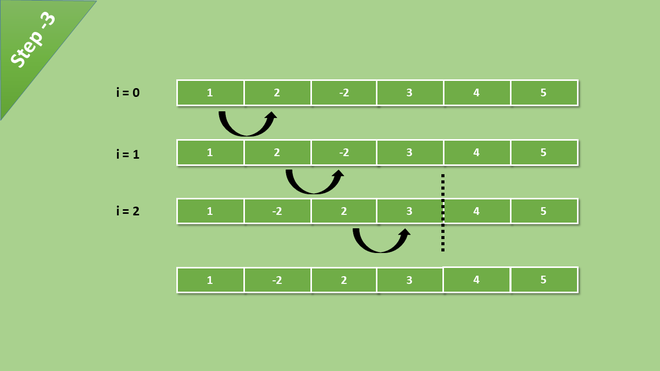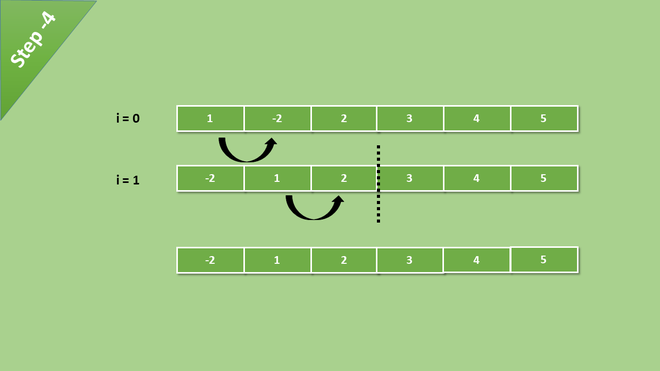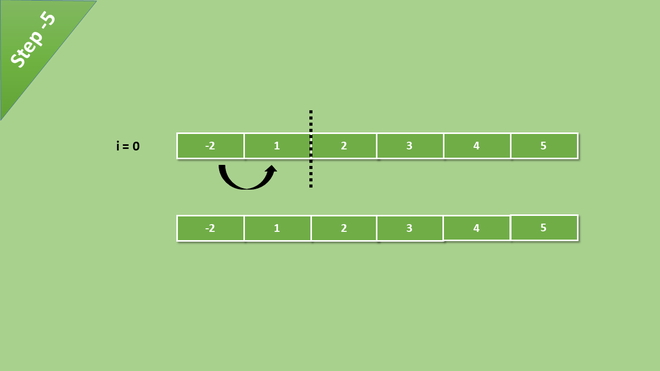After all the iteration and comparisons of elements, we get a sorted array.

## Syntax

```BubbleSort(array){
for i -> 0 to arrayLength
for j -> 0 to (arrayLength - i - 1)
if arr[j] > arr[j + 1]
swap(arr[j], arr[j + 1])
}```

## Javascript

 `// Bubble sort Implementation using Javascript`` ` ` ` `// Creating the bblSort function`` ``function` `bblSort(arr){``    ` ` ``for``(``var` `i = 0; i < arr.length; i++){``    ` `   ``// Last i elements are already in place  ``   ``for``(``var` `j = 0; j < ( arr.length - i -1 ); j++){``      ` `     ``// Checking if the item at present iteration ``     ``// is greater than the next iteration``     ``if``(arr[j] > arr[j+1]){``        ` `       ``// If the condition is true then swap them``       ``var` `temp = arr[j]``       ``arr[j] = arr[j + 1]``       ``arr[j+1] = temp``     ``}``   ``}`` ``}`` ``// Print the sorted array`` ``console.log(arr);``}`` ` ` ` `// This is our unsorted array``var` `arr = [234, 43, 55, 63,  5, 6, 235, 547];`` ` ` ` `// Now pass this array to the bblSort() function``bblSort(arr);`
```Output
Sorted array :
[5, 6, 43, 55, 63, 234, 235, 547]```

Note: This implementation is not optimized. We will see the optimized solution next.

## Optimized Solution

As we discussed the implementation of bubble sort earlier that is not optimized. Even If the array is sorted, the code will run with O(n^2) complexity. Let’s see how to implement an optimized bubble sort algorithm in javascript.

### The syntax for Optimized solution

```BubbleSort(array){
for i -> 0 to arrayLength
isSwapped <- false
for j -> 0 to (arrayLength - i - 1)
if arr[j] > arr[j + 1]
swap(arr[j], arr[j + 1])
isSwapped -> true
}```

## Javascript

 `// Optimized implementation of bubble sort Algorithm`` ` `function` `bubbleSort(arr){``   ` `  ``var` `i, j;``  ``var` `len = arr.length;``   ` `  ``var` `isSwapped = ``false``;``   ` `  ``for``(i =0; i < len; i++){``     ` `    ``isSwapped = ``false``;``     ` `    ``for``(j = 0; j < len; j++){``        ``if``(arr[j] > arr[j + 1]){``          ``var` `temp = arr[j]``          ``arr[j] = arr[j+1];``          ``arr[j+1] = temp;``          ``isSwapped = ``true``;``        ``}``    ``}``     ` `    ``// IF no two elements were swapped by inner loop, then break ``     ` `    ``if``(!isSwapped){``      ``break``;``    ``}``  ``}``   ` `  ``// Print the array``  ``console.log(arr)``}`` ` ` ` `var` `arr = [243, 45, 23, 356, 3, 5346, 35, 5];`` ` `// calling the bubbleSort Function``bubbleSort(arr)`
```Output
Sorted Array :
[3, 5, 23, 35, 45, 243, 356, 5346]```

## Complexities

#### Worst Case and Average case time complexity

If the array is in reverse order then this condition is the worst case and Its time complexity is O(n2).

#### Best case time complexity

If the array is already sorted then it is the best-case scenario and its time complexity is O(n)

Auxiliary Space: O(1)

My Personal Notes arrow_drop_up